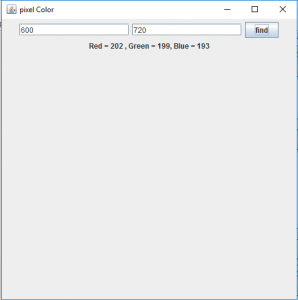# Java Robot Class | Get the pixel Color of a given point

Robot is part of java.awt package . Robot class is basically used to generate native system input events for the purposes of self- running demos, test automation, and other application where control over mouse and keyboard is used.
Robot class generates events that can be used to control mouse, keyboard and can be used to take screenshot of the screen.
In this article we will discuss how to get the pixel color of the point on the screen mentioned by the user.

Method used :

```getPixelColor(int x, int y)
This function returns an object of the color class
of the given screen coordinates. ```

In the following program we will print a label that will contain the RGB values of the pixel entered and the text of the label will be of the pixel Color

 `// Java program to get the pixel color of ` `// given screen coordinates ` `import` `java.awt.*; ` `import` `javax.swing.*; ` `import` `java.awt.event.*; ` `public` `class` `colour ``extends` `JFrame ` `    ``implements` `ActionListener { ` `    ``// textfield to get x, y coordinates ` `    ``static` `JTextField x, y; ` ` `  `    ``// button ` `    ``static` `JButton b; ` ` `  `    ``// create a frame ` `    ``static` `JFrame f; ` ` `  `    ``// label ` `    ``static` `JLabel l; ` ` `  `    ``public` `static` `void` `main() ` `    ``{ ` ` `  `        ``// create a frame ` `        ``f = ``new` `JFrame(``"pixel Color"``); ` ` `  `        ``// label to show the RGB value ` `        ``l = ``new` `JLabel(``"no value"``); ` ` `  `        ``// create the text field ` `        ``x = ``new` `JTextField(``16``); ` `        ``y = ``new` `JTextField(``16``); ` ` `  `        ``// create a button ` `        ``b = ``new` `JButton(``"find"``); ` ` `  `        ``// create an object of the class ` `        ``colour co = ``new` `colour(); ` ` `  `        ``// add ActionListener ` `        ``b.addActionListener(co); ` ` `  `        ``// create a panel ` `        ``JPanel p = ``new` `JPanel(); ` ` `  `        ``// add textfield and utton to the panel ` `        ``p.add(x); ` `        ``p.add(y); ` `        ``p.add(b); ` `        ``p.add(l); ` ` `  `        ``// add the panel ` `        ``f.add(p); ` ` `  `        ``// set the size of the frame ` `        ``f.setSize(``500``, ``500``); ` `        ``f.show(); ` `    ``} ` ` `  `    ``// if the button is pressed ` `    ``public` `void` `actionPerformed(ActionEvent e) ` `    ``{ ` `        ``String s = e.getActionCommand(); ` `        ``if` `(s.equals(``"find"``)) { ` `            ``int` `xp, yp; ` ` `  `            ``// get user inputs of x and y position ` `            ``xp = Integer.parseInt(x.getText()); ` `            ``yp = Integer.parseInt(y.getText()); ` ` `  `            ``// try and catch block to handle exceptions ` `            ``try` `{ ` `                ``// create an object of robot class ` `                ``Robot r = ``new` `Robot(); ` ` `  `                ``// get the pixel color ` `                ``c = r.getPixelColor(xp, yp); ` `            ``} ` `            ``catch` `(Exception evt) { ` `                ``// print error message ` `                ``System.err.println(evt.getMessage()); ` `            ``} ` ` `  `            ``Color c; ` ` `  `            ``// set the RGB value to the label ` `            ``// and to its foreground ` `            ``l.setForeground(c); ` `            ``l.setText(``"Red = "` `+ c.getRed() + ",  ` `Green = ``" + c.getGreen() + "``, Blue = " + c.getBlue()); ` `        ``} ` `    ``} ` `} `

Output :Note : the following program might not run in an online compiler please use an offline IDE.

Attention reader! Don’t stop learning now. Get hold of all the important Java and Collections concepts with the Fundamentals of Java and Java Collections Course at a student-friendly price and become industry ready.

My Personal Notes arrow_drop_upThird year Department of Information Technology Jadavpur University

If you like GeeksforGeeks and would like to contribute, you can also write an article using contribute.geeksforgeeks.org or mail your article to contribute@geeksforgeeks.org. See your article appearing on the GeeksforGeeks main page and help other Geeks.

Please Improve this article if you find anything incorrect by clicking on the "Improve Article" button below.

Article Tags :
Practice Tags :

Be the First to upvote.

Please write to us at contribute@geeksforgeeks.org to report any issue with the above content.